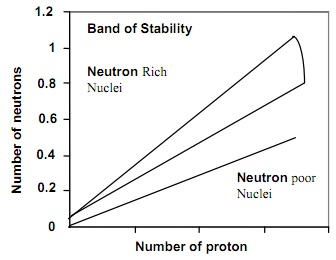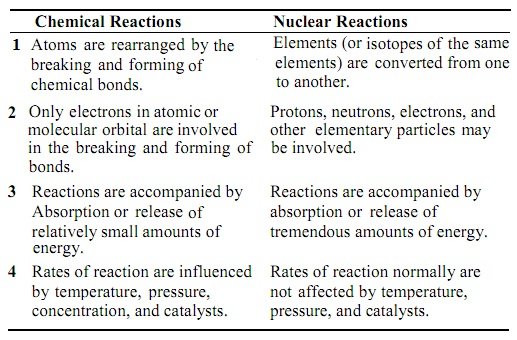Introduction:

Radioactivity is the phenomenon in which some elements discharge small particles known as radiation, to form the other element. This is in contrary to the accepted Dalton postulate of indestructibility of an atom. Though, only elements having unstable nuclei termed as radioactive are capable of undergoing the natural radioactivity whereas stable nuclei do not. All the elements having atomic number more than 83 are radioactive and they experience nuclear transmutation (that is, nuclear reaction) which differ considerably from ordinary chemical reactions.

The knowledge of radioactivity has contributed vastly to medicine, industry, archeology, scientific research, engineering and agriculture.

With the exception of hydrogen (11H), each and every atom of an element consists of a nucleus that comprises of neutrons and protons, having electrons occupying significant empty space around the nucleus. The nucleus is a minute fraction of net volume of atom, yet almost all the mass of an atom resides in the nucleus. Therefore, nuclei are extremely dense. It has been illustrated experimentally that nucleus of all the elements has around the similar density of 2.4 x 1014g/cm3. Table below illustrates the general properties of fundamental components of an element.

Table: Basic Constituents of an Atomic Element

Particle             Mass                    Charge

Electron (e-)    0.00054858 amu       -1

Proton (P)       1.0073 amu               +1

Neutron (n)     1.0087 amu               None

The ratio of number of neutron to proton in any nucleus verifies the stability of the nucleus and as well affect nuclear reaction is termed as neutron-proton ratio.

The neutron-proton ratio of an element states which if element will naturally undergoes the radioactivity or not.

Neutron-Proton Ratio and Nuclear Stability:

The main factor which finds out the stability of nucleus is the neutron-proton ratio (n/p). A nucleus is stated to be stable if it doesn't experience radioactivity. Around 275 various nuclei have no proof of radioactive decay, therefore they are very stable. 157 nuclides out of it have even number of proton and even number of neutron (that is, even-even nuclei), 52 nuclides have even number of proton and odd number neutron. 50 nuclides have odd number of protons and even number of neutron whereas just four nuclides have odd number of both proton and neutrons. Such are represented in the table shown below:

Table:  Abundance of naturally occurring Nuclides

Number of particles          Even/Odd   Even/Odd    Even/Odd    Even/Odd

Number of protons                Even        Even             Odd           Odd

Number of neutrons               Even        Odd             Even          Odd

Number of such nuclides        157           50                52               4

The given are rules which guide the prediction of the nuclear stability:

A)  Nuclides having 'Magic number' (2, 8, 20, 28, 50, 126) of protons or a number of neutrons or a sum of the two have unusual stability.

B)  Nuclei having even number of both protons and neutrons are usually more stable than those having odd numbers of these particles

C)  All the isotopes of the elements having atomic numbers higher than 83 are radioactive.

The figure below is a plot of the number of neutrons (N) versus number of protons (Z) for the stable nuclides (that is, the band of stability). For low atomic numbers, the most stable nuclides encompass equivalent numbers of protons and neutrons (N - Z). Above atomic number 20, the most stable nuclides encompass more neutrons as compare to protons. Careful examination reveals an about stepwise shape to the plot because of the stability of nuclides having even numbers of nucleons.Fig: Number of neutrons vs number of protons for stable nuclides

Nuclei whose neutron-to-proton (n/p) ratios lie outside the stable region go through spontaneous radioactive decay by emitting one or more particles and /or electromagnetic rays. Based on whether the nucleus is above, below or to the right of the band of stability (Figure shown above), it emits different kinds of particles in a nuclear reaction.  Note that in a nuclear reaction, changes of one particle to other take place in a pattern which exhibits that nuclear reaction distinct from ordinary chemical reaction as summarized in table below:

Table: Comparison of Chemical Reactions and Nuclear ReactionsBoth the chemical and nuclear reactions are depicted via a complete chemical equation. Though, two main rules guide writing the chemical equation of nuclear reaction:

a) The sum of the mass numbers of the reactants should be equivalent the sum of mass number of the products.

b) The sum of atomic number of the reactions should be equivalent to the atomic number of the products, this keep charge balance.

Some of the nuclei are unstable; therefore they emit sub-atomic particles or electromagnetic radiation in a phenomenon termed as radioactivity. Radioactivity can be of two kinds namely, natural and artificial radioactivity.

Natural Radioactivity:  This is a kind of radioactivity that takes place spontaneously, emitting electromagnetic radiation and particles that comprise beta, positron and alpha particles. The occurrence of this kind of decay or emission takes place, based on the position of nuclei whether above, below or sides of the stability/belt region. Most of the naturally radioactive nuclei lie outside this belt.

Artificial radioactivity: This is a non-spontaneous form of radioactivity that needs effect of bombardment of the nuclei having sub-atomic particles. It is or else termed as anthropogenic or induced radioactivity.

Juliot and his wife, Irene Curie discovered artificial radioactivity in the year 1934 in which aluminum nuclei is bombarded with He nuclei to form new element having emission of electromagnetic ray or particles:

42He + 2713Al → 3014P + 11n

Particles Emission and Position of Stable Region:

Above the Band of Stability: Neutron-Rich Region

The nuclei in this area encompass a very high ratio of neutron to protons than those in the belt.  To reduce this ratio and move down toward the belt of stability, they go through a nuclear reaction termed as Beta particle emission. The beta particle is an electron ejected from the nucleus whenever a neutron is transformed to a proton.

10n → 11P + 0-1β

The emission of Beta particle leads to an increase in the number of proton in the nucleus and a simultaneous reduce in the number of neutrons.

Classical illustrations:

22888Ra → 22889Ac + 0-1β

9740Zr → 9741Nb + 0-1β

4019K → 4020Ca + 0-1β

Below the Band of Stability: Neutron-Poor Region

The nuclei here encompass lower neutron-to-proton ratios than those in the band thus they require increasing this ratio and afterward moving up toward the belt of stability. The nuclei undergo two possible kinds of nuclear reaction; positron emission or electron capture (K capture).

a) Position emission: This is most generally encountered with artificially radioactive nuclei of the higher element. Classical illustrations are:

iiP → 10n + 0+1β

3819K → 3818Ar + 0+1β

b)  Electron captures (K capture): This is a capture of an electron generally a 1s electron through the nucleus. The captured electron joins by a proton to form a neutron in such a way that the atomic number reduces by one whereas the mass number remains the same; therefore it consists of the similar net effect as positron emission. Classical illustrations:

3718Ar + 0-1e → 3717Cl

10647Ar 0-1e → 10646Pd

This is significant to note that some of the neutrons-poor nuclei, specifically the heavier ones, raise their neutron-to-proton ratios by undergoing alpha emission. The alpha particle is emitted from nucleus thus reducing the mass number by 4 and atomic number by 2.

Nuclei having Atomic number greater than 83:

All the elements having atomic numbers higher than 83, are radioactive in nature. They undergo a nuclear reaction via emitting alpha particles (α). Classical illustrations are:

22688Ra → 22284e + 42α

21084Po → 20184Pb + 42α

Gamma Ray Emission:

The gamma rays are high energy radiation, emitted whenever an unstable nucleus experiences a rearrangement of its constituent particle to provide more stable, lower energy nucleus. Gamma rays are often emitted all along with other kind of subatomic particles.

9943Tc → 9943Tc + 00γ

It will be noted that pure gamma emitters are rare, however instead, the radiation gamma accompany either alpha or beta radiation.

Tutorsglobe: A way to secure high grade in your curriculum (Online Tutoring)